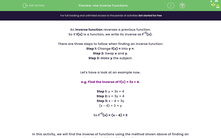# Use Inverse Functions

In this worksheet, students find the inverse of functions using the method of finding an alternative subject.Key stage:  KS 4

Year:  GCSE

GCSE Subjects:   Maths

GCSE Boards:   Pearson Edexcel, OCR, Eduqas, AQA,

Curriculum topic:   Algebra

Curriculum subtopic:   Notation, Vocabulary and Manipulation Language of Functions

Difficulty level:#### Worksheet Overview

An inverse function reverses a previous function.

So if f(x) is a function, we write its inverse as f-1(x).

There are three steps to follow when finding an inverse function:

Step 1: Change f(x) = into y =.

Step 2: Swap x and y.

Step 3: Make y the subject.

Let's have a look at an example now.

e.g. Find the inverse of f(x) = 3x + 4.

Step 1: y = 3x + 4

Step 2: x = 3y + 4

Step 3: x - 4 = 3y

(x - 4) ÷ 3 = y

So f-1(x) = (x - 4) ÷ 3

In this activity, we will find the inverse of functions using the method shown above of finding an alternative subject of the function.

### What is EdPlace?

We're your National Curriculum aligned online education content provider helping each child succeed in English, maths and science from year 1 to GCSE. With an EdPlace account you’ll be able to track and measure progress, helping each child achieve their best. We build confidence and attainment by personalising each child’s learning at a level that suits them.

Get started